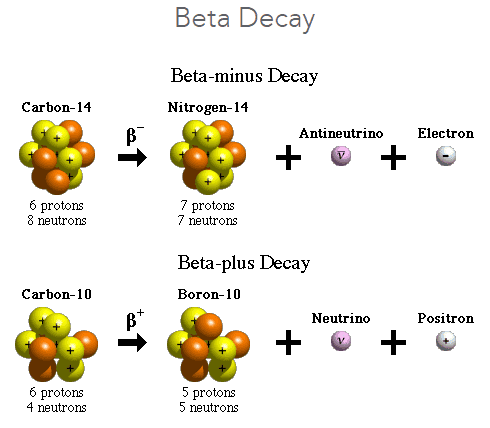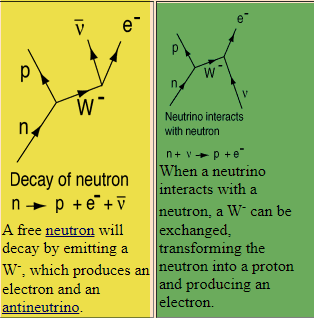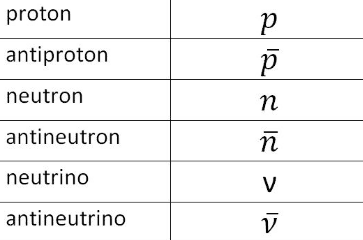# Beta decay and the Boson

• I
• PainterGuy

#### PainterGuy

TL;DR Summary
I was trying to understand beta decay as a beginner but came across some difficult point and need your guidance. Thank you!
Hi,

Please note that I'm trying to understand it at very basic level. I'd really appreciate if you could help me with the queries below.

Under the section The weak force it says:
The weak force, also called the weak nuclear interaction, is responsible for particle decay. This is the literal change of one type
of subatomic particle into another. So, for example, a neutrino that strays close to a neutron can turn the neutron into a proton while the neutrino becomes an electron.

Physicists describe this interaction through the exchange of force-carrying particles called bosons. Specific kinds of bosons are responsible for the weak force, electromagnetic force and strong force. In the weak force, the bosons are charged particles called W and Z bosons. When subatomic particles such as protons, neutrons and electrons come within 10^-18 meters, or 0.1% of the diameter of a proton, of one another, they can exchange these bosons. As a result, the subatomic particles decay into new particles

In nuclear physics and particle physics, the weak interaction, which is also often called the weak force or weak nuclear force, is one of the four known fundamental interactions, with the others being electromagnetism, the strong interaction, and gravitation. It is the mechanism of interaction between subatomic particles that is responsible for the radioactive decay of atoms.
Source: https://en.wikipedia.org/wiki/Weak_interaction

The following excerpt is from Wikipedia article on Beta decay, https://en.wikipedia.org/w/index.php?title=Beta_decay&oldid=1028366739.
In nuclear physics, beta decay (β-decay) is a type of radioactive decay in which a beta particle (fast energetic electron or positron) is emitted from an atomic nucleus... For example, beta decay of a neutron transforms it into a proton by the emission of an electron accompanied by an antineutrino; or, conversely a proton is converted into a neutron by the emission of a positron with a neutrino in so-called positron emission.

Question 1:
In case of beta decay, a neutron converts into a proton along with an electron and antineutrino; i.e. beta-minus decay. But where does the $W^{-}$ boson come from? The neutron doesn't seem to interact with any other particle, so it means that there is no boson present and hence no weak force interaction. Where am I going wrong?By the way, similar question could be found here: https://physics.stackexchange.com/q...sons-in-the-weak-nuclear-force-and-beta-decay

In my opinion, first two answers seem to imply that there exists two different mechanism for the beta decay. One is natural where a neutron decays on its own and there is another mechanism where a neutrino interacts with neutron and weak force plays its role thru exchange of a boson and neutron decays.

Question 2(i):
Just as the electromagnetic force is transmitted by means of carrier particles known as photons, the weak force is exchanged via three types of intermediate vector bosons.
Source: https://www.britannica.com/science/W-particle

I understand that both photon and boson are force carrying particles but they are very much different at the same time. Bosons have comparative huge mass while photons are massless. Boson breaks down into other particles such as electron and antineutrino. Please check the part in yellow highlight below.Source: http://hyperphysics.phy-astr.gsu.edu/hbase/Forces/funfor.html

Question 2(ii):
To me boson looks more like an intermediary unstable particle rather than a particle carrying any force. For example, looking at the part of picture in yellow highlight above, it seems like neutron decays naturally into a proton by emitting a W- boson which further breaks down into electron and antineutrino. Looking at the part of picture in green highlight, it looks like when neutrino and neutron come in close contact, it produces proton and another unstable particle W- is emitted which breaks down further. Do I make any sense?

Question 3:
The neutron discovered in may 1932.

In 1933, Enrico Fermi proposed the first theory of the weak interaction, known as Fermi's interaction. He suggested that beta decay could be explained by a four-fermion interaction, involving a contact force with no range... The existence of the W and Z bosons was not directly confirmed until 1983.
Source: https://en.wikipedia.org/wiki/Weak_interaction#History

In a famous letter written in 1930, Wolfgang Pauli attempted to resolve the beta-particle energy conundrum by suggesting that, in addition to electrons and protons, atomic nuclei also contained an extremely light neutral particle, which he called the neutron. He suggested that this "neutron" was also emitted during beta decay (thus accounting for the known missing energy, momentum, and angular momentum), but it had simply not yet been observed. In 1931, Enrico Fermi renamed Pauli's "neutron" the "neutrino" ('little neutral one' in Italian). In 1933, Fermi published his landmark theory for beta decay, where he applied the principles of quantum mechanics to matter particles, supposing that they can be created and annihilated, just as the light quanta in atomic transitions. Thus, according to Fermi, neutrinos are created in the beta-decay process, rather than contained in the nucleus; the same happens to electrons. The neutrino interaction with matter was so weak that detecting it proved a severe experimental challenge. Further indirect evidence of the existence of the neutrino was obtained by observing the recoil of nuclei that emitted such a particle after absorbing an electron. Neutrinos were finally detected directly in 1956 by Clyde Cowan and Frederick Reines in the Cowan–Reines neutrino experiment. The properties of neutrinos were (with a few minor modifications) as predicted by Pauli and Fermi.
Source: https://en.wikipedia.org/wiki/Beta_decay#Neutrinos

I think Fermi was trying to explain Beta(-) decay which produces antineutrino. Fermi was using his four fermion interaction theory. So, I think what he was postulating as neutrino was in fact antineutrino. Do I have it correct?

The antineutrino discovered around 1956 by Cowan and Reines is the antiparticle of the electron neutrino.

Positron and also beta(+) decay was observed in 1934.

Proton, neutron, electron, and neutrino are fermions. The following table shows commonly used symbols for the particles.1: https://en.wikipedia.org/wiki/Positron_emission#Discovery_of_positron_emission
2: https://physics.stackexchange.com/questions/142303/what-is-the-weak-force
3: https://arxiv.org/ftp/arxiv/papers/1803/1803.07147.pdf
4: https://en.wikipedia.org/wiki/Cowan–Reines_neutrino_experiment
5: https://en.wikipedia.org/wiki/Positron_emission#Discovery_of_positron_emission

•vanhees71
1. There is no emitted W. The W is an intermediate virtual particle.

2. You are taking the diagrams too literally. They are really pictographical representations of a mathematical expression and the W is an internal line, meaning that it is an intermediate virtual particle - never a produced particle. The W mass is way too high for it to be produced as a real particle in beta decays.

•PainterGuy, Astronuc, vanhees71 and 1 other person
As @Orodruin already stated, forget about the popular-science mumbling about "virtual particles" to begin with. Also don't think in forces, which is a non-relativistic concept, but in "interactions".

The classical analogy you should use to think about relativistic QT is indeed the field picture. Already in the classical case we describe interactions not as forces, acting instantaneously at a distance as was conjectured (with some explicitly expressed doubts!) by Newton concerning the gravitational force, but in a local way using fields. According to this picture, discovered by Faraday in an intuitive way based on his careful experiments, e.g., the electromagnetic interaction is described via fields. This means that around a moving electrically charged particle there's inevitably an electromagnetic field. Due to this field a force is acting on a particle determined by the value of the electromagnetic field at the momentary position of this particle.

It now turns out that the only successful relativistic QT is described in terms of quantized fields and also the locality of interactions (microcausality) is a fundamental ingredient. In this picture everything is unified in terms of quantized fields. Both the "matter" or "particles" and the fields describing the interaction between particles are described by quantum fields. Non-interacting quantum fields also have a kind or particle interpretation, i.e., certain states of the fields can be interpreted as particles.

Usually we cannot solve for the equations governing the relativistic QFTs exactly but we rely on perturbation theory, i.e., a formal expansion of the time-evolution operator in powers of the coupling constants, and the corresponding formulae for these calculations are very effectively written in terms of the Feynman diagrams.

The formalism also precisely tells us how to interpret them in terms of physical phenomena: Only (and really only) the external legs of the Feynman diagrams represent "particles", i.e., the specific states of free quantum fields (the socalled one-particle Fock states). The physical picture behind this is that what we want to calculate are transition probabilities of scattering processes: You have (usually two) particles in the initial state. We can consider them being prepared so far from each other that the interactions between them can be neglected, and for such (and only such) asymptotic free states there's a particle interpretation possible. Now you shoot the particles toward each other, so that they come close. Then the interaction becomes important, and there's no particle interpretation possible anymore and rather we have think in terms of fields again. The scattering of two charged particles in quantum electrodynamics is, at lowest order perturbation theory, described by the electromagnetic field. The Feynman diagram for elastic scattering consists of two incoming charged particles and two outgoing charged particles (asymptotic free states) connected by a curly line representing the electromagnetic field (in terms of the socalled propagator of this field). So there is no "virtual particle" but just an electromagnetic field.

This works also for the other interactions (except gravity yet). The weak interaction is mediated by the socalled W- and Z-boson fields. E.g., ##\beta## decay on a fundamental level describes the decay of a down quark (one of the constituents of the neutron, which is a complicated bound state due to the strong interaction of two down and one up quarks) to an up quark, an electron, and an anti-electron-neutrino. The curly line connecting the one incoming down-quark line and the three particles (u-quark, electron, anti-electron-neutrino) stands for a ##W^-##-field propagator.

In contradistinction to the photons in QED the W and Z bosons are unstable, i.e., they cannot be prepared as asymptotic free states but have to be inferred from interacting particles which can be prepared in such asymptotic free states. E.g., the Z-boson can be discovered checking the elastic scattering of an electron and a positron at high energies. It turns out that these leptons do not only interact via the electromagnetic but also via the weak interaction, i.e., in addition to the Feynman diagram with an internal photon line (depicting a propgator of the electromagnetic field) there's also a Feynman diagram with an internal Z-boson line (depicting a propagator of the Z-field), and this gives rise to a bump in the corresponding cross section.

•PainterGuy, weirdoguy, Astronuc and 1 other person
Summary:: I was trying to understand beta decay as a beginner but came across some difficult point and need your guidance. Thank you!

In case of beta decay, a neutron converts into a proton along with an electron and antineutrino; i.e. beta-minus decay. But where does the W boson come from?
Let me try to help out here. I myself asked similar question a while ago here and I think the best answer I got was that first of all the W+- and Z bosons come from theory. And the closest we have ever been to them is by studying the particle interactions at CERN where after many many proton beam collisions scientists studied the newly formed particles their trajectories and energies and found that the theorized particles explain what they saw. In reality those as you yourself said "intermediary" particles never even make it to the detectors outside the proton beam vacuum tube because of the short lived nature of them. The whole interaction and birth of new particles within the proton - proton collisions is so fast that it doesn't matter whether they are physically there or not , you would be unable to detect them anyway.

It's like the Fast and Furious movie, the W and Z bandits (I mean bosons) they are gone before the "particle police" can detect them.

Live fast and die young gets a whole new meaning with the W and Z bosons.

•PainterGuy
Just to add some thoughts - this phenomena where the W bosons "only exist formally" and are never directly observed happens all the time; for instance you never observe the Higgs Boson either; only its decay products. But, by looking at the distribution of the observed decay products (e.g. one of the first channels probed for the Higgs was Higgs -> two photons), you can infer the existence of the Higgs, even if in some sense it "only exists in the math".

In the particular case of the W boson, since (as others have mentioned) the W boson is very off-shell in these processes you describe, you can actually write down an effective theory where the W boson doesn't show up at all, and all you have is a four-fermion interaction
https://en.wikipedia.org/wiki/Fermi's_interaction
(in fact historically, this was the *original* theory I believe, before they realized W bosons existed). You only really start to "see" the W boson when you have energetic-enough processes. This is again a common theme - for example the stout believers of supersymmetry will tell you that we have to keep going to higher and higher energies to hopefully resolve the super partners that should exist